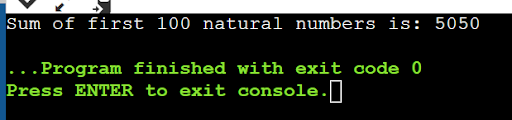Write a program in C++, to print the sum of first 100 natural numbers using for control structure.

Write a program in C++, to print the sum of first 100 natural numbers using for control structure.

verified

#include <iostream>

using namespace std;

int main()

{

int i, sum=0;

for(i=1;i<=100;i++)

{

sum=sum+i;

}

cout<<"Sum of first 100 natural numbers is: "<<sum;

return 0;

}

Output::